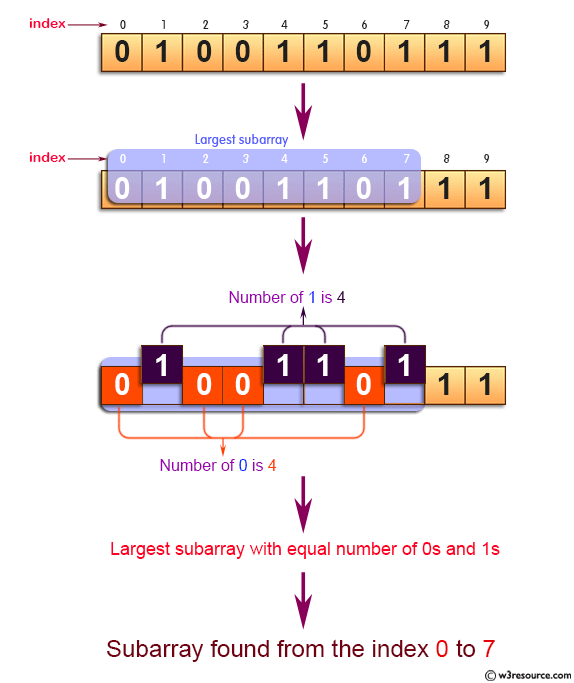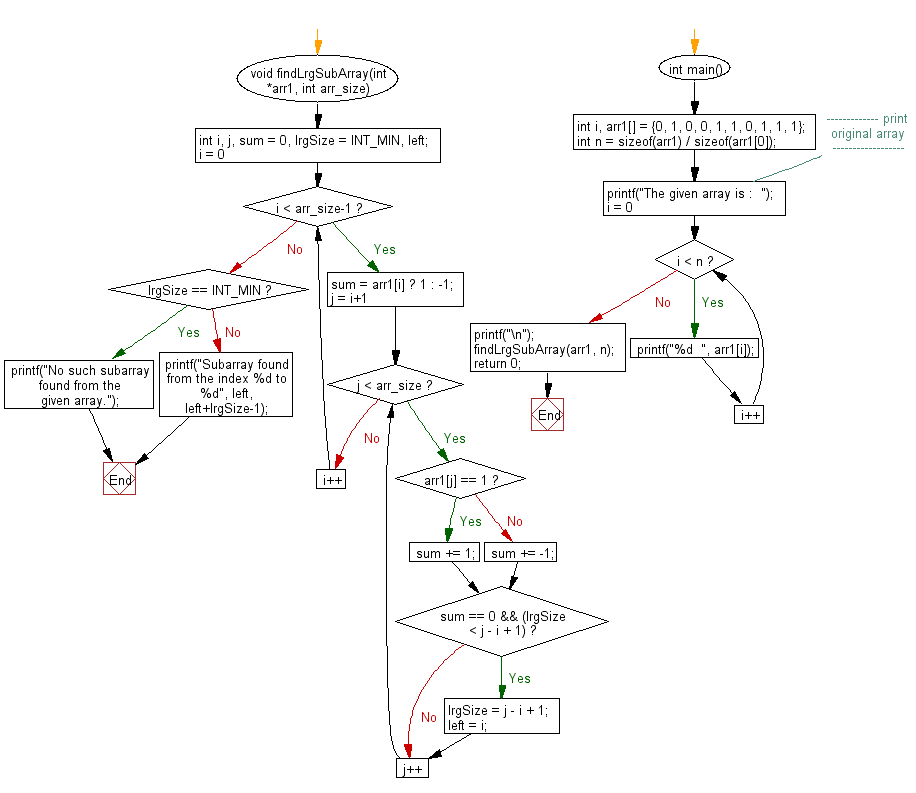﻿ C exercises: Find the largest subarray with equal number of 0s and 1s - w3resource# C Exercises: Find the largest subarray with equal number of 0s and 1s

## C Array: Exercise-62 with Solution

Write a program in C to find the largest subarray with equal number of 0s and 1s.

Pictorial Presentation:Sample Solution:

C Code:

``````#include <stdio.h>
#include <limits.h>

void findLrgSubArray(int *arr1, int arr_size)
{
int i, j, sum = 0, lrgSize = INT_MIN, left;

for(i = 0; i < arr_size-1; i++)
{
sum = arr1[i] ? 1 : -1;
for (j = i+1; j < arr_size; j++)
{
if (arr1[j] == 1)
sum += 1;
else
sum += -1;
if (sum == 0 && (lrgSize < j - i + 1))
{
lrgSize = j - i + 1;
left = i;
}
}
}

if (lrgSize == INT_MIN)
{
printf("No such subarray found from the given array.");
} else
{
printf("Subarray found from the index %d to %d", left, left+lrgSize-1);
}
}

int main()
{
int i, arr1[] = {0, 1, 0, 0, 1, 1, 0, 1, 1, 1};
int n = sizeof(arr1) / sizeof(arr1);
//------------- print original array ------------------
printf("The given array is :  ");
for(i = 0; i < n; i++)
{
printf("%d  ", arr1[i]);
}
printf("\n");
//------------------------------------------------------
findLrgSubArray(arr1, n);
return 0;
}
```
```

Sample Output:

```The given array is :  0  1  0  0  1  1  0  1  1  1
Subarray found from the index 0 to 7
```

Flowchart:C Programming Code Editor:

Improve this sample solution and post your code through Disqus.

What is the difficulty level of this exercise?

﻿